Cairo's compositing operators

Normally, you will be using cairo to draw objects on top of each other. But cairo can do differently, if you need it! In fact, you can use all the Porter/Duff compositing operators.

Fourteen different operators are available since cairo 1.0; more operators have been added in cairo 1.10. This page is a try to describe them. It may contain errors and is possibly incomplete. Please help to improve it!

# Example images

It is probably best to describe the effect of compositing operators by showing example images, so that's what is done below. The images show the result of drawing an object (a translucent blue rectangle) onto a surface which already contains another image (a translucent red rectangle).

The operator names try to describe what the operators mean. Two words need to be mentioned: the source and the destination (or dest). In this context, these provide the two objects which contribute to the graphical output of the drawing operation. The destination is the name of the surface before the drawing operation, the source is the name of what is added while drawing.

## The Destination

To create the example images, the red rectangle is first drawn to the (empty) destination surface with the default operator:

``````cairo_rectangle (cr, 0, 0, 120, 90);
cairo_set_source_rgba (cr, 0.7, 0, 0, 0.8);
cairo_fill (cr);
``````

The surface is now a transparent plane with a partially transparent rectangle in it. This represents the destination to which the next drawing operation will be performed. Both the red and the fully transparent part of the surface are now natural parts of the destination.

## Setting the compositing operator

The current operator is now set using `cairo_set_operator()`.

## The Source

The tutorial describes how drawing works in cairo:

"Cairo internally draws with one fundamental drawing operation: the source and mask are freely placed somewhere over the destination. Then the layers are all pressed together and the paint from the source is transferred to the destination wherever the mask allows it."

However, there is no mention of a mask in the names of the compositing operators. They refer to a source and a destination only. This allows for two different interpretations with regard to what should be regarded as the source for the compositing operation:

1. Since the mask determines where the paint from the source is transferred, it also determines the area in which the compositing operation is performed. This interpretation can be called bounded.

2. The area where the compositing operation is performed is not bounded by the mask. Instead, any parts where the source layer is not transferred are considered to be fully transparent. We call this interpretation of the source unbounded.

For some of the operators, both interpretations lead to the same result, for others they do not. In cairo, the actual interpretation depends on the operator. The unbounded operators modify destination outside of the bounds of the mask. However, their effect can still be limited by way of clipping. See the section on clipping and masking for those details.

The blue rectangle is drawn with the new operator in effect:

``````cairo_rectangle (cr, 40, 30, 120, 90);
cairo_set_source_rgba (cr, 0, 0, 0.9, 0.4);
cairo_fill (cr);
``````

The results can be seen in the images below. Note that both rectangles are drawn with transparency. When studying the images, you may be able to guess how they would look for opaque objects, too. For reference, the code to create the images is also available.

# Operators

For the mathematical description, it is convenient to use the following conventions:

1. The index A (assigned to color components and transparency factor) refers to the source, while the index B refers to the destination. The index R refers to the result of the compositing operation.

2. Each pixel in both the source and the destination is fully described by a tuple (r, g, b, a). We will use "x" as a shorthand for any color component, since they are always treated the same. "a" describes the alpha value (opacity) and is treated differently.

3. The product of xA and aA is described by xaA. xaB and xaR have analogous meaning.

The color computations equations contain a division by aR, which cannot be done where aR is zero. But in this case the color doesn't matter anyway (full transparency) and can also be set to zero. (Internally, the colors may be handled differently, so this might or might not be necessary.)Where the second object is drawn, the first is completely removed. Anywhere else it is left intact. The second object itself is not drawn.

The effect of the `CLEAR` operator depends on the interpretation of the source. In cairo, this operator is bounded.

 Resulting alpha (aR) Resulting color (xR) 0 0

## CAIRO_OPERATOR_SOURCE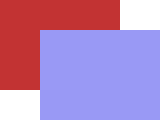The second object is drawn as if nothing else were below. Only outside of the blue rectangle the red one is left intact.

The effect of the `SOURCE` operator depends on the interpretation of the source. In cairo, this operator is bounded.

 Resulting alpha (aR) Resulting color (xR) aA xA

## CAIRO_OPERATOR_OVERThe image shows what you would expect if you held two semi-transparent slides on top of each other. This operator is cairo's default operator.

The output of the `OVER` operator is the same for both bounded and unbounded source.

 Resulting alpha (aR) Resulting color (xR) aA + aB·(1−aA) (xaA + xaB·(1−aA))/aR

## CAIRO_OPERATOR_INThe first object is removed completely, the second is only drawn where the first was.

Note that the transparency of the first object is taken into account. That is, the small blue rectangle is slightly lighter than it would have been, had the first object been opaque.

The effect of the `IN` operator depends on the interpretation of the source. In cairo, this operator is unbounded.

 Resulting alpha (aR) Resulting color (xR) aA·aB xA

## CAIRO_OPERATOR_OUT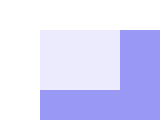The blue rectangle is drawn only where the red one wasn't. Since the red one was partially transparent, you can see a blue shadow in the overlapping area. Otherwise, the red object is completely removed.

The effect of the `OUT` operator depends on the interpretation of the source. In cairo, this operator is unbounded.

 Resulting alpha (aR) Resulting color (xR) aA·(1−aB) xA

## CAIRO_OPERATOR_ATOP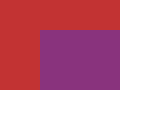This leaves the first object mostly intact, but mixes both objects in the overlapping area. The second object object is not drawn except there.

If you look closely, you will notice that the resulting color in the overlapping area is different from what the `OVER` operator produces. Any two operators produce different output in the overlapping area!

The output of the `ATOP` operator is the same for both bounded and unbounded source.

 Resulting alpha (aR) Resulting color (xR) aB xaA + xB·(1−aA)

## CAIRO_OPERATOR_DEST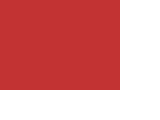Leaves the first object untouched, the second is discarded completely.

The output of the `DEST` operator is the same for both bounded and unbounded source.

 Resulting alpha (aR) Resulting color (xR) aB xB

## CAIRO_OPERATOR_DEST_OVER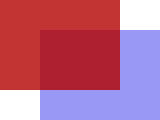The result is similar to the `OVER` operator. Except that the "order" of the objects is reversed, so the second is drawn below the first.

The output of the `DEST_OVER` operator is the same for both bounded and unbounded source.

 Resulting alpha (aR) Resulting color (xR) (1−aB)·aA + aB (xaA·(1−aB) + xaB)/aR

## CAIRO_OPERATOR_DEST_INThe blue rectangle is used to determine which part of the red one is left intact. Anything outside the overlapping area is removed.

This works like the `IN` operator, but again with the second object "below" the first.

Similar to the two interpretations of the source, it is possible to imagine the same distinction with regard to the destination. In cairo, the `DEST_IN` operator is unbounded.

 Resulting alpha (aR) Resulting color (xR) aA·aB xB

## CAIRO_OPERATOR_DEST_OUTThe second object is used to reduce the visibility of the first in the overlapping area. Its transparency/opacity is taken into account. The second object is not drawn itself.

The output of the `DEST_OUT` operator is the same for both bounded and unbounded interpretations.

 Resulting alpha (aR) Resulting color (xR) (1−aA)·aB xB

## CAIRO_OPERATOR_DEST_ATOP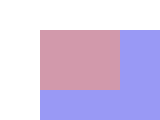Same as the `ATOP` operator, but again as if the order of the drawing operations had been reversed.

In cairo, the `DEST_ATOP` operator is unbounded.

 Resulting alpha (aR) Resulting color (xR) aA xA·(1−aB) + xaB

## CAIRO_OPERATOR_XOR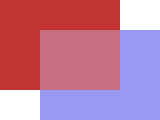The output of the `XOR` operator is the same for both bounded and unbounded source interpretations.

 Resulting alpha (aR) Resulting color (xR) aA + aB − 2·aA·aB (xaA·(1−aB) + xaB·(1−aA))/aRThe output of the `ADD` operator is the same for both bounded and unbounded source interpretations.

 Resulting alpha (aR) Resulting color (xR) min(1, aA+aB) (xaA + xaB)/aR

## CAIRO_OPERATOR_SATURATEThe output of the `SATURATE` operator is the same for both bounded and unbounded source interpretations.

 Resulting alpha (aR) Resulting color (xR) min(1, aA+aB) (min(aA, 1−aB)·xA + xaB)/aR

# Blend Modes

Since cairo 1.10, the set of operators is extended by 15 additional operators. These originate from the PDF specification and extend the range of available color computation equations. All of them share the same alpha computation equation, which is identical to the one from `CAIRO_OPERATOR_OVER`. The output of the new operators is the same for both bounded and unbounded source.

The color computations are more complicated than for the previous operators, so a different presentation is used for the mathematical details. The resulting alpha (aR) is:

aR = aA + aB·(1−aA)

The equation for the color components follows this form:

xR = 1/aR · [ (1−aB)·xaA + (1−aA)·xaB + aA·aB·f(xA,xB) ]

where the operators differ only in the blend mode function f(xA,xB). The respective blend mode functions used are given below. The result color is undefined when aR is zero (full transparency).

## CAIRO_OPERATOR_MULTIPLY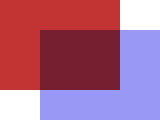Blend mode function:

f(xA,xB) = xA·xB

The result color is at least as dark as the darker of the two input colors.

## CAIRO_OPERATOR_SCREEN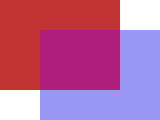Blend mode function:

f(xA,xB) = xA + xB − xA·xB

Input colors are complemented and multiplied, the product is complemented again. The result is at least as light as the lighter of the input colors.

## CAIRO_OPERATOR_OVERLAYBlend mode function:

if (xB ≤ 0.5) f(xA,xB) = 2·xA·xB else f(xA,xB) = 1 − 2·(1 − xA)·(1 − xB)

Multiplies or screens colors, depending on the lightness of the destination color.

## CAIRO_OPERATOR_DARKENBlend mode function:

f(xA,xB) = min(xA,xB)

Selects the darker of the color values in each component.

## CAIRO_OPERATOR_LIGHTENBlend mode function:

f(xA,xB) = max(xA,xB)

Selects the lighter of the color values in each component.

## CAIRO_OPERATOR_COLOR_DODGE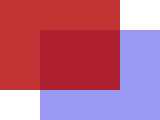Blend mode function:

if (xA < 1) f(xA,xB) = min(1, xB/(1−xA)) else f(xA,xB) = 1

Brightens the destination color by a factor depending on the source color.

## CAIRO_OPERATOR_COLOR_BURNBlend mode function:

if (xA > 0) f(xA,xB) = 1 − min(1, (1−xB)/xA) else f(xA,xB) = 0

Darkens the destination color by a factor depending on the source color.

## CAIRO_OPERATOR_HARD_LIGHT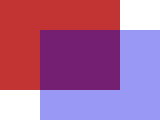Blend mode function:

if (xA ≤ 0.5) f(xA,xB) = 2·xA·xB else f(xA,xB) = 1 − 2·(1 − xA)·(1 − xB)

Multiplies or screens colors, depending on the lightness of the source color.

## CAIRO_OPERATOR_SOFT_LIGHT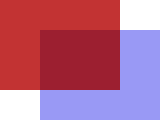Blend mode function:

if (xA ≤ 0.5) f(xA,xB) = xB − (1 − 2·xA)·xB·(1 − xB) else f(xA,xB) = xB + (2·xA − 1)·(g(xB) − xB)

where

if (x ≤ 0.25) g(x) = ((16·x − 12)·x + 4)·x else g(x) = sqrt(x)

Darkens or lightens, depending on the source color.

## CAIRO_OPERATOR_DIFFERENCEBlend mode function:

f(xA,xB) = abs(xB−xA)

Takes the difference of the destination and source colors.

## CAIRO_OPERATOR_EXCLUSIONBlend mode function:

f(xA,xB) = xA + xB − 2·xA·xB

The effect is similar to `DIFFERENCE`, but has lower contrast.

# Non-separable Blend Modes

The following blend modes are non-separable, i.e., they consider all color components in combination. The blend mode functions `f(xA,xB)` become `f(cA,cB)`. A few more functions are necessary to describe them. "`c`" is the color defined by the tuple (r,g,b).

The original functions are provided in the PDF specfication (see Links below).

sat(c) := max(r,g,b) − min(r,g,b) lum(c) := 0.3·r + 0.59·g + 0.11·b clip_color(c) := { L = lum(c) N = min(r,g,b) X = max(r,g,b) if N < 0.0 r = L + (((r − L)·L)⁄(L − N)) g = L + (((g − L)·L)⁄(L − N)) b = L + (((b − L)·L)⁄(L − N)) if X > 1.0 r = L + (((r − L)·(1 − L))⁄(X − L)) g = L + (((g − L)·(1 − L))⁄(X − L)) b = L + (((b − L)·(1 − L))⁄(X − L)) return c } set_lum(c, L) := { D = L − lum(c) r = r + D g = g + D b = b + D return clip_color(c) }

In the following function "`cmax`" and "`cmin`" serve as aliases for the colors with the highest and lowest values. "`cmid`" is the third (remaining) color.

set_sat(c, S) := { if (cmax > cmin) cmid = (((cmid − cmin)·S)⁄(cmax − cmin)) cmax = S else cmid = cmax = 0.0 cmin = 0.0 return c }

## CAIRO_OPERATOR_HSL_HUEBlend mode function:

f(cA,cB) = set_lum(set_sat(cA, sat(cB)), lum(cB))

Creates a color with the hue of the source and the saturation and luminosity of the destination.

## CAIRO_OPERATOR_HSL_SATURATIONBlend mode function:

f(cA,cB) = set_lum(set_sat(cB, sat(cA)), lum(cB))

Creates a color with the saturation of the source and the hue and luminosity of the destination. Painting with this mode onto a gray area produces no change.

## CAIRO_OPERATOR_HSL_COLOR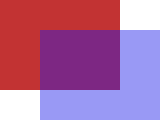Blend mode function:

f(cA,cB) = set_lum(cA, lum(cB))

Creates a color with the hue and saturation of the source and the luminosity of the destination. This preserves the gray levels of the destination and is useful for coloring monochrome images or tinting color images.

## CAIRO_OPERATOR_HSL_LUMINOSITY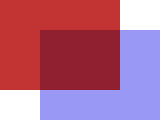Blend mode function:

f(cA,cB) = set_lum(cB, lum(cA))

Creates a color with the luminosity of the source and the hue and saturation of the destination. This produces an inverse effect to `CAIRO_OPERATOR_HSL_COLOR`.

This section augments the documentation of cairo's compositing model by describing how clipping and masking are taken into account when rendering. In this context the clip and mask are treated as cairo surfaces with alpha content that both default to full opaqueness when not explicitly given. The basic approach to clipping in cairo is to first perform the operation without clipping and then interpolating by the clip pointwise between the unclipped result and the destination. In the descriptions below this is achieved using a ternary operator A LERPT B that is defined here as:

A LERPT B := (A IN T) ADD (B OUT T)

The approach to masking is to combine it using operator IN with either the clip, for the bounded operators, or with the source for the unbounded ones.

There are three distinct rendering equations used within cairo to implement clipping and masking, which can be described using the (unclipped, unmasked) operators IN, OUT and ADD operators as primitives.

Kind Equation
XRender ((src IN mask) OP dst) LERPclip dst
Bounded (src OP dst) LERP(clip IN mask) dst
Simple (src IN (clip IN mask)) OP dst

The XRender-kind definition most closely matches the letter of the XRender specification as it reduces to XRender's equation when clip is binary valued – the only kind of clip talked about in the spec. The Simple-kind definition is the fastest to execute and was conceived of as an optimisation of the XRender-kind definition to take advantage of the fact that for many operators the two coincide. The Bounded definition is a variation of the XRender-kind definition where the mask is considered to be a part of the clip. It is used for the SOURCE and CLEAR operators only, and is used to make those operators be explicitly bounded by mask. The following table gives the rendering equation used by each of cairo's operators. The entries marked with Any are operators for which all three variants coincide.

Operator Kind
CLEAR Bounded
SOURCE Bounded
OVER Any
IN XRender
OUT XRender
ATOP Any
DEST Any
DEST_OVER Any
DEST_IN XRender
DEST_OUT Any
DEST_ATOP XRender
XOR Any